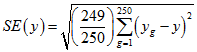4720.0 - National Aboriginal and Torres Strait Islander Social Survey: User Guide, 2014-15
Latest ISSUE Released at 11:30 AM (CANBERRA TIME) 27/05/2016
 Page tools: .mffeedback,#pjs { display :none; } Enable Javascript to Print PagesPrint AllEmail NotificationRSSSearch this Product Abbreviations Replicate weights technique (Technical Note) REPLICATE WEIGHTS TECHNIQUE Overview 1 A class of techniques called 'replication methods' provide a general process of estimating variances for the types of complex sample designs and weighting procedures employed in ABS household surveys. 2 The basic idea behind the replication approach is to select sub-samples repeatedly from the whole sample. For each of these sub-samples the statistic of interest is calculated. The variance of the full sample statistic is then estimated using the variability among the replicate statistics calculated from these sub-samples. The sub-samples are called 'replicate groups' and the statistics calculated from these replicates are called 'replicate estimates'. 3 There are various ways of creating replicate sub-samples from the full sample. The replicate weights produced for the 2014–15 National Aboriginal and Torres Strait Islander Social Survey (NATSISS) have been created under the delete-a-group jack-knife method of replication which is described below. 4 Some of the advantages in using replicate weights are that: the same process is applicable to most statistics, such as means, percentages, ratios, correlations, derived statistics and regression coefficients; and it is not necessary to have detailed survey design information, if the replicate weights are included with the survey data file. Derivation of replicate weights 5 The delete-a-group jack-knife method of replicate weighting was used to derive weights, through the following process: 250 replicate groups were formed with each group formed to mirror the overall sample. Units from a cluster of dwellings all belong to the same replicate group, and a unit can belong to only one replicate group; for each replicate weight, one replicate group was omitted from the weighting and remaining records were weighted in the same manner as for the full sample; the records in that group that was omitted received a weight of zero; this process was repeated for each replicate group (i.e. a total of 250 times); and each record had 250 replicate weights attached to it with one of these being the zero weight. Application of replicate weights 6 As noted above, replicate weights enable variances of estimates to be calculated relatively simply. They also enable unit record analyses such as chi-square and logistic regression to be conducted, which take into account the sample design. 7 Replicate weights for any variable of interest can be calculated from the 250 replicate groups, giving 250 replicate estimates. The distribution of this set of replicate estimates, in conjunction with the full sample estimate (based on the general weight) is then used to approximate the variance of the full sample. 8 The formulae for calculating the Standard error (SE), relative standard error (RSE) and 95% Margin of Error (MoE) of an estimate using this method is shown below.where: g = (1, ..., 250) (the number of replicate weights); y(g) = estimate from using replicate weighting; and y = estimate from using full person weight. 9 The RSE(y) = SE(y)/y*100. 10 The 95% MoE(y)=SE(y)*1.96. 11 This method can also be used when modelling relationships from unit record data, regardless of the modelling technique used. In modelling, the full sample would be used to estimate the parameter being studied, such as a regression co-efficient. The 250 replicate groups would be used to provide 250 replicate estimates of the survey parameter. The variance of the estimate of the parameter from the full sample is then approximated, as above, by the variability of the replicate estimates. Availability of RSEs calculated using replicate weights 12 Actual RSEs were used in the summary publication released from this survey. The RSEs for estimates published in the National Aboriginal and Torres Strait Islander Social Survey, 2014–15 (cat no. 4714.0) are available in spreadsheet format from the Downloads tab. The RSEs in the spreadsheets were calculated using the replicate weights methodology. Availability of MoEs calculated using replicate weights 13 Actual MoEs for proportion estimates have been calculated in the summary publication released from this survey. The MoEs for estimates published in the National Aboriginal and Torres Strait Islander Social Survey, 2014–15 (cat no. 4714.0) are available in spreadsheet format from the Downloads tab. The MoEs in the spreadsheets were calculated using the replicate weights methodology. Reliability of estimates 14 For further information relating to the reliability of estimates, including the calculation of relative standard errors, margins of error, comparison of proportions, significance testing, and age standardisation, see Technical Note: Reliability of estimates from the National Aboriginal and Torres Strait Islander Social Survey, 2014–15 (cat no. 4714.0). Document Selection These documents will be presented in a new window.